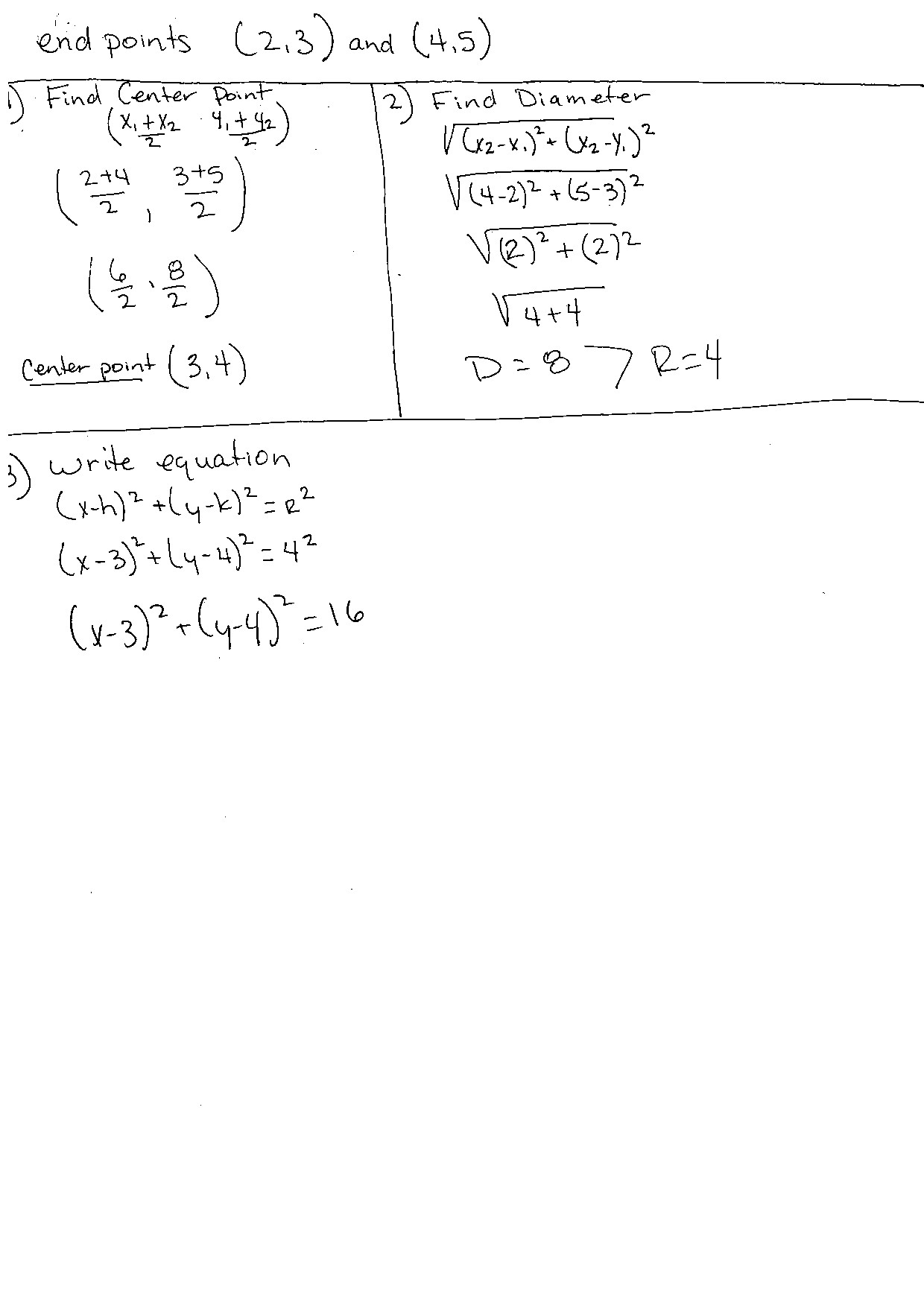# end points (2,3) and (4.5)A Find Center Point.X, + X2Find Diameter4,+ 422.3+5Vi4-2)= + (s-3)²Ve)'+(2)2+ (5-3)24+4Center point (3,4)D=87R=4write equation3)(xrh)" +(y-k)²= e²(x-3)*+Ly-1)* = 4²(v-3}"-Cy4)*= 16

Question
76 views

I am stuck on an equation and can't figure out at what step I am going wrong. I would appreciate some step by step and formula by formula assistance, please!!!! I have attached my work for this question. Please see attachement

Find the equation with a diameter whose end points are (2,3) and (4,5)

Thank,

Iliciahelp_outlineImage Transcriptioncloseend points (2,3) and (4.5) A Find Center Point. X, + X2 Find Diameter 4,+ 42 2. 3+5 Vi4-2)= + (s-3)² Ve)'+(2)2 + (5-3)2 4+4 Center point (3,4) D=87R=4 write equation 3) (xrh)" +(y-k)²= e² (x-3)*+Ly-1)* = 4² (v-3}"-Cy4)*= 16 fullscreen
check_circle

Step 1

Given that, the end points of the diameter of a circle is,

Step 2

The center point of the circle is computed as follows.

Step 3

### Want to see the full answer?

See Solution

#### Want to see this answer and more?

Solutions are written by subject experts who are available 24/7. Questions are typically answered within 1 hour.*

See Solution
*Response times may vary by subject and question.
Tagged in

### Equations and In-equations